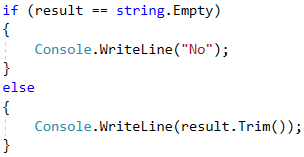# Problem: Five Special Letters

Two numbers are given: start and end. Write a program that generates all combinations of 5 letters, each among the sets of {'a', 'b', 'c', 'd', 'e'} so that the weight of these 5 letters is a number in the range [start … end], inclusive. Print them in alphabetical order, in a single row, separated by a space.

The weight of the letters is calculated as follows:

weight('a') = 5;
weight('b') = -12;
weight('c') = 47;
weight('d') = 7;
weight('e') = -32;


The weight of the sequence of the letters c1, c2, …, cn is calculated by removing all the letters that are repeated (from right to left) and then calculating the formula:

weight(c1c2…cn) = 1 * weight(c1) + 2 * weight(c2) + … + n * weight(cn)


For example, the weight of bcddc is calculated as follows:

First, we remove the repeating letters and get bcd. Then we apply the formula: 1 * weight('b') + 2 * weight('c') + 3 * weight('d') = 1 * (-12) + 2 * 47 + 3 * 7 = 103.

Another example: weight("cadae") = weight("cade") = 1 * 47 + 2 * 5 + 3 * 7 + 4 * (-32) = -50.

## Input Data

The input data is read from the console. It consists of two numbers:

• The number for start.
• The number for end.

Input data will always be valid and will always be in the format described. No need to check.

## Output Data

The result should be printed on the console as a sequence of strings, arranged in alphabetical order. Each string must be separated from the next one by a single space. If the weight of any of the 5 letter strings does not exist within the specified range, print "No".

## Constraints

• Numbers for start and end are integers in the range [-10000 … 10000].
• Allowed program time: 0.25 seconds.
• Allowed memory: 16 MB.

## Sample Input and Output

40
42
weight("bdcea") = 40
Input Output
-1
1
Input Output
200
300
Input Output
300
400
No

## Hints and Guidelines

Let’s give some hints and guidelines for solving this problem.

As every problem, we start the solution by reading and processing the input data. In this case, we have two integers that can be processed with a combination of the int.Parse(…) and Console.ReadLine() methods.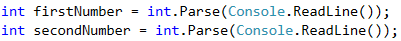We have several main points in the problem – generating all combinations with a length of 5 including the 5 letters, removing repeating letters and calculating weight for a simplified word. The answer will consist of every word whose weight is within the given range [firstNumber, secondNumber].

### Generating All Combinations

In order to generate all combinations with length of 1 using 5 symbols, we would use a loop from 0 to 4, as we want each number of the loop to match one character. In order to generate any combinations of length 2 using 5 characters (i.e. "aa", "ab", "ac", …, "ba", …), we would create two nested loops each running through the digits from 0 to 4, as we will once again make sure that each digit matches a specific character. We will repeat this step 5 times, so we will finally have 5 nested loops with indexes i1, i2, i3, i4 and i5.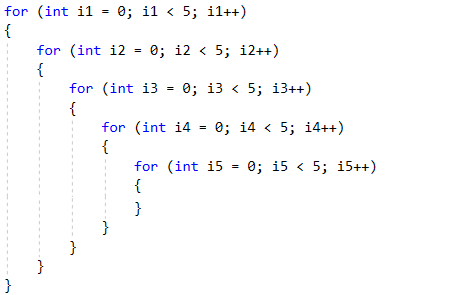### Transforming Combinations into Words

Now that we have all 5-digit combinations, we must find a way to "turn" the five digits into a word with the letters from 'a' to 'e'. One of the ways to do that is to predefine a simple string that contains the letters that we have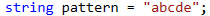and for each digit we take the letter from the particular position. This way, the number 00000 will become "aaaaa", and the number 02423 will become "acecd". We can create the 5-letter string in the following way.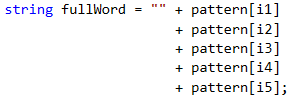Another way: we can convert the digits to letters by using their arrangement in the ASCII table. The expression 'a' + i return the result 'a' in case i = 0, 'b' in case i = 1, 'c' in case i = 2, etc.

This way we already have generated all 5-letter combinations and can proceed with the following part of the task.

Attention: as we have chosen a 'pattern' that takes into consideration the alphabetical arrangement of the letters, and cycles are run in the appropriate manner, the algorithm will generate the works in alphabetical order and there is no need for additional sorting before printing the output.

### Removing Repetitive Letters

Once we have the finished string, we have to remove all the repeating symbols. We will do this by adding the letters from left to right in a new string and each time before adding a letter, we will check if it already exists – if it does, we will skip it and if it doesn't, we will add it. To begin with, we will add the first letter to the starting string.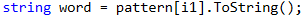Then we will do the same with the other 4, checking each time with the following condition and the .IndexOf(…) method. This can be done with a loop by fullWord (leaving it to the reader for exercise), and it can be done in the lazy way by copy-paste.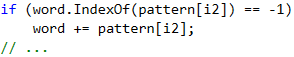The .IndexOf(…) method returns the index of the particular element if it is found or -1 if the item is not found. Therefore, every time we get -1, it means that we still do not have this letter in the new string with unique letters and we can add it, and if we get a value other than -1, this will mean we already have the letter and we'll not add it.

### Calculating Weight

Calculating the weight is simply going through the unique word (word) obtained in the last step, and for each letter we need to take its weight and multiply it by the position. For each letter, we need to calculate what value we will multiply its position by, for example by using a switch construction.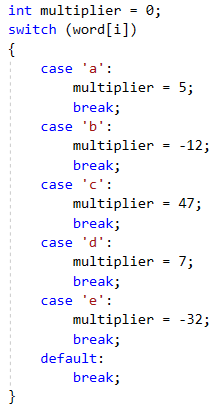Once we have the value of that letter, we should multiply it by its position. Because the indexes in the string differ by 1 from the actual positions, i.e. index 0 is position 1, index 1 is position 2, etc., we will add 1 to the indexes.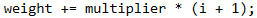All intermediate results obtained must be added to the total amount for each letter of the 5-letter combination.

### Preparing the Output

Whether a word needs to be printed is determined by its weight. We need a condition to determine if the current weight is in the range [start … end] passed to the input at the start of the program. If this is the case, we print the full word (fullWord).

Be careful not to print the word with unique letters. It was only needed to calculate the weight!

The words are separated with a space and we'll accumulate them in an intermediate variable result, which is defined as an empty string at the beginning.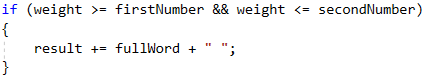### Final Touches

The condition is met unless we do not have a single word in the entered range. In order to find out if we have found a word, we can simply check whether the string result has its initial value (i.e., an empty string), if it does, we print No, otherwise we print the whole string without the last space (using the .Trim ()) method.